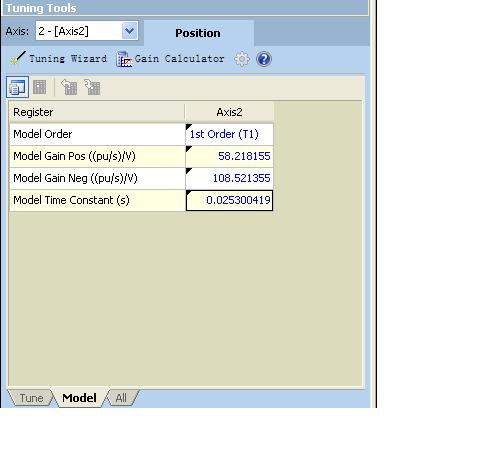# what's the meaning of model parameter?

what’s the meaning of model parameter? I can not find the Detailed explanation。

model gain pos、model gain neg、model time constantThe autotuning calculates the mathematical model of the system. The parameters it calculates are standard mathematical concepts. A first order system is defined by a gain and a time constant. The gain is in units of ((pu/s)/V). This tells us how fast the axis moves for each volt of Control Output. There is a gain for each direction. The time constant defines how quickly the axis responds. The small time constant means the axis responds quickly.